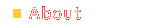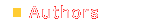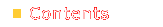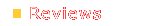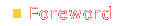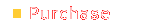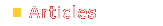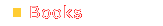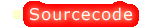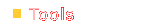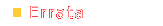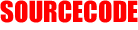``` crackerbox# nmap -vv -sS -O www.example.org Starting Nmap V. 3.00 by fyodor@insecure.org (www.insecure.org/Nmap) Host www.example.org (10.5.10.20) appears to be up ... good. Initiating SYN half-open stealth scan against www.example.org (10.5.10.20) The SYN scan took 1 second to scan 1525 ports. For OSScan assuming that port 22 is open and port 1 is closed and neither are firewalled Interesting ports on www.example.org (10.5.10.20) (The 1518 ports scanned but not shown below are in state: closed) Port State Service 22/tcp open ssh 25/tcp open smtp 515/tcp open printer 6000/tcp open X11 TCP Sequence Prediction: Class=random positive increments Difficulty=3728145 (Good luck!) Sequence numbers: FA401E9 FA401E9 F720DEB F720DEB 1004486A 1004486A Remote operating system guess: Linux 2.1.122 - 2.2.16 OS Fingerprint: TSeq(Class=RI%gcd=1%SI=38E311) T1(Resp=Y%DF=Y%W=7F53%ACK=S++%Flags=AS%Ops=MENNTNW) T2(Resp=N) T3(Resp=Y%DF=Y%W=7F53%ACK=S++%Flags=AS%Ops=MENNTNW) T4(Resp=Y%DF=N%W=0%ACK=O%Flags=R%Ops=) T5(Resp=Y%DF=N%W=0%ACK=S++%Flags=AR%Ops=) T6(Resp=Y%DF=N%W=0%ACK=O%Flags=R%Ops=) T7(Resp=Y%DF=N%W=0%ACK=S%Flags=AR%Ops=) PU(Resp=Y%DF=N%TOS=C0%IPLEN=164%RIPTL=148%RID=E%RIPCK=E%UCK=E%ULEN=134%DAT=) Nmap run completed -- 1 IP address (1 host up) scanned in 0 seconds ```